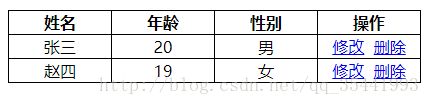# Uncaught TypeError: Failed to execute ‘appendChild’ on ‘Node’: parameter 1 is not of type ‘Node How to Fix

I want to dynamically insert a line of data into tBody, and I write the following code:

``````html:
<table id="theList">
<th>姓名</th>
<th>年龄</th>
<th>性别</th>
<th>操作</th>
<tbody id="myBody"></tbody>
</table>

js:

let list = []
let temp = []

list.push({
name: '张三',
age: 20,
sex: '男'
})
list.push({
name: '赵四',
age: 19,
sex: '女'
})
function render(data) {
var html=[]
for (let i = 0; i < data.length; i++) {
let template = '<tr><td>'+data[i].name+'</td><td>'+data[i].age+'</td><td>'+data[i].sex+'</td><td><a href="javascript:;">修改</a>&nbsp;&nbsp;<a href="javascript:;">删除</a></td></tr>'
html.push(template)
document.getElementById('myBody').appendChild(html.join(''))
}
}
render(list)
``````

The browser throws an Uncaught TypeError when running the above code: Failed to execute ‘appendChild’ on ‘Node’ : parameter 1 is not of type ‘Node’. To find out the cause of the error by looking up data:
AppendChild () requires that a tr object be passed in, not a tr string
and html.join(“) above is a string

``console.log(typeof html.join(''))  //stirng``

Solution:
render function

``````function render(data) {
for (let i = 0; i < data.length; i++) {
let tr = document.createElement('tr')
tr.innerHTML = '<td>'+data[i].name+'</td><td>'+data[i].age+'</td><td>'+data[i].sex+'</td><td><a href="javascript:;">修改</a>&nbsp;&nbsp;<a href="javascript:;">删除</a></td>'
document.getElementById('myBody').appendChild(tr)
}
}``````

Tr is an object. Instead of writing like this, you might as well just write:

``````function render(data) {
var html=[]
for (let i = 0; i < data.length; i++) {
let template = '<tr><td>'+data[i].name+'</td><td>'+data[i].age+'</td><td>'+data[i].sex+'</td><td><a href="javascript:;">修改</a>&nbsp;&nbsp;<a href="javascript:;">删除</a></td></tr>'
html.push(template)
document.getElementById('myBody').innerHTML = html.join('')
}
}``````

Operation effect:Other features are still being implemented… .